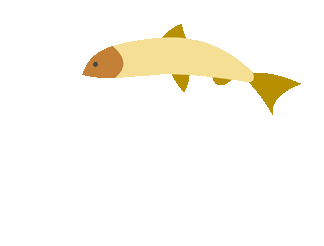## Math Notes

These are notes that have been created by your teacher.

So, it is the same language and vocabulary that you were exposed to in class.##### Place Value Notes

Topics that fall under the numbers and operations category are as follows: Place Value with Whole Numbers, Place Value with Decimals, and Comparing Numbers##### Operations Notes

Topics that fall under the numbers and operations category are as follows: Adding and Subtracting Whole Numbers, Multiplying Whole Numbers and Decimals, Dividing Whole Numbers and Decimals.##### Fractions Notes

Topics that fall under the numbers and operations category are as follows: Prime and Composite Numbers, GCF and LCM, and last but not least, Fractions!##### Algebra Notes

Topics that fall under the algebra category are as follows: Order of Operations, Evaluating Expressions, Solving Equations, and Inequalities.##### Geometry Notes

Topics that fall under the geometry category are as follows: Perimeter and Area, Geometric Solids, and Volume.##### Data Notes

Topics that fall under the data category are as follows: Graphs, Coordinate System, and Central Tendancy (Mean, Median, Mode, and Range)